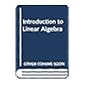Normal view

## Introduction to higher algebra / Maxime Bôcher

Material type:TextLanguage: English Publisher: New York : Macmillan, 1929Description: xi, 321 páginas ; 21 x 15 cmContent type: texto Media type: sin mediación Carrier type: volumenSubject(s): ÁlgebraDDC classification: 512.5
Contents:
Polynomials and their most fundamental properties -- A few properties of determinants -- The theory of linear dependence -- Linear equations -- Some theorems concerning the rank of a matrix -- Linear transformations and the combination of matrices -- Invariants. First Principles and ilustrations -- Bilinear forms -- Geometric introduction to the study of quadratic forms -- Quadratic forms -- Real quadratic forms -- The system of a quadratic form and one or more linear forms -- Pairs of quadratic forms -- Some properties of polynomials in general -- Factors and common factors of polynomials in one variable and of binary forms -- Factors of polynomials in two or more variables -- General theorems on integral rational invariants -- Symmetric polynomials -- Polynomials symmetric in pairs of variables -- Elementary divisors and the equivalence of λ-Matrices -- The equivalence and classification of pairs of bilinear forms and of collineations -- The equivalence and classification of pairs of quadratic forms
Tags from this library: No tags from this library for this title.Average rating: 0.0 (0 votes)
Item type Current location Call number Status Date due Barcode Item holdsLibros BIBLIOTECA DE CIENCIAS
512.5 B364 (Browse shelf) Available 121134
Total holds: 0
##### Browsing BIBLIOTECA DE CIENCIAS shelves Close shelf browser
 No cover image availableNo cover image available No cover image available No cover image available No cover image available 512.5 A159 Linear and multilinear algebra / 512.5 A545 Introduction to linear algebra / 512.5 An634i Introducción al álgebra lineal / 512.5 B364 Introduction to higher algebra / 512.5 B486 Dual algebras with applications to invariant subspaces and dilation theory / 512.5 C136 v1 Algèbre linéaire 1 / 512.5 C136 v1 Algèbre linéaire 1 /

Polynomials and their most fundamental properties -- A few properties of determinants -- The theory of linear dependence -- Linear equations -- Some theorems concerning the rank of a matrix -- Linear transformations and the combination of matrices -- Invariants. First Principles and ilustrations -- Bilinear forms -- Geometric introduction to the study of quadratic forms -- Quadratic forms -- Real quadratic forms -- The system of a quadratic form and one or more linear forms -- Pairs of quadratic forms -- Some properties of polynomials in general -- Factors and common factors of polynomials in one variable and of binary forms -- Factors of polynomials in two or more variables -- General theorems on integral rational invariants -- Symmetric polynomials -- Polynomials symmetric in pairs of variables -- Elementary divisors and the equivalence of λ-Matrices -- The equivalence and classification of pairs of bilinear forms and of collineations -- The equivalence and classification of pairs of quadratic forms